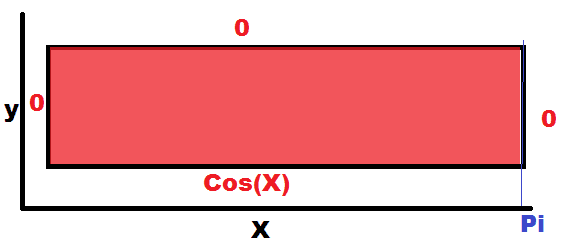# Partial Differentiation Laplace Equation Question

## Homework Statement

Consider the Laplace Equation of a semi-infinite strip such that 0<x< π and y>0, with the following boundary conditions:
\begin{equation}
\frac{\partial u}{\partial x} (0, y) = \frac{\partial u}{\partial x} (0,\pi) = 0
\end{equation}

\begin{equation}
u(x,0) = cos(x) \text{for}\ 0<x<\pi
\end{equation}

\begin{equation}
u(x,y) = \to 0 \ \text{as} \ y \to 0 \ \text{for}\ 0<x<\pi
\end{equation}

## Homework Equations

General Solution:
\begin{equation}
\begin{split}
u(x,y) = \frac{a_0}{2L}(L-y) + \sum_{n = 1}^\infty \alpha_n sinh(\frac{n\pi}{L})(L-y)cos(\frac{n\pi x}{L}) \\
\text{where} \ \alpha_n = \frac{a_n}{sinh(n\pi} \\
\text{where} \ a_n = \frac{2}{L} \int_0^L cos(\frac{n\pi x}{L}) f(x) dx
\end{split}
\end{equation}

## The Attempt at a Solution

I am asking if I setup the problem right so far.
The visualization (i.e my picture of how I visualize the problem) The boundary conditions.My attempt so far:
\begin{equation}
u_{xx} + u_{yy} = 0
\end{equation}

Assume:
\begin{equation}
u = F(x)G(y)
\end{equation}

Thus:
\begin{equation}
\begin{split}
u_{xx} = F''(x)G(y) \\
u_{yy} = F(x)G''(y) \\
F''(x)G(y) + F(x)G''(y) = 0 \\
\end{split}
\end{equation}
From this, we can define the following:
\begin{equation}
\frac{F''(x)}{F(x)} = \frac{G''(y)}{G(y)} = K \ \text{some constant}
\end{equation}
If u(x,0) = cos(x)
\begin{equation}
F(x)G(0) = cos(x) \\
\end{equation}
Can I assume that G(y) is equal to sin(y)?

Last edited:

Ray Vickson
Homework Helper
Dearly Missed

## Homework Statement

Consider the Laplace Equation of a semi-infinite strip such that 0<x< π and y>0, with the following boundary conditions:
\begin{equation}
\frac{\partial u}{\partial x} (0, y) = \frac{\partial u}{\partial x} (0,\pi) = 0
\end{equation}

\begin{equation}
u(x,0) = cos(x) \text{for}\ 0<x<\pi
\end{equation}

\begin{equation}
u(x,y) = \to 0 \ \text{as} \ y \to 0 \ \text{for}\ 0<x<\pi
\end{equation}

## Homework Equations

General Solution:
\begin{equation}
\begin{split}
u(x,y) = \frac{a_0}{2L}(L-y) + \sum_{n = 1}^\infty \alpha_n sinh(\frac{n\pi}{L})(L-y)cos(\frac{n\pi x}{L}) \\
\text{where} \ \alpha_n = \frac{a_n}{sinh(n\pi} \\
\text{where} \ a_n = \frac{2}{L} \int_0^L cos(\frac{n\pi x}{L}) f(x) dx
\end{split}
\end{equation}

## The Attempt at a Solution

I am asking if I setup the problem right so far.
The visualization (i.e my picture of how I visualize the problem) The boundary conditions.No: you said that ##u_x(0,y) = 0## and ##u_x(0,\pi) = 0##: I assume you want ##u_x(0,y) = u_x(\pi,y) = 0##. If so, your boundary conditions in the diagram are incorrect: you do not need to have ##u = 0## on the vertical sides; you need ##u_x = 0## on the sides.

Furthermore, you say that ##u(x,0) = \cos x## for ##0 < x < \pi##, and you also say ##u(x,y) \to 0## as ##y \to 0##. These contradict each other; which do you mean?

No: you said that ##u_x(0,y) = 0## and ##u_x(0,\pi) = 0##: I assume you want ##u_x(0,y) = u_x(\pi,y) = 0##. If so, your boundary conditions in the diagram are incorrect: you do not need to have ##u = 0## on the vertical sides; you need ##u_x = 0## on the sides.

Furthermore, you say that ##u(x,0) = \cos x## for ##0 < x < \pi##, and you also say ##u(x,y) \to 0## as ##y \to 0##. These contradict each other; which do you mean?
Yes, I want ##u_x (\pi,y) = 0,## sorry for the confusion. And also, I meant to write as ##y \to \infty##